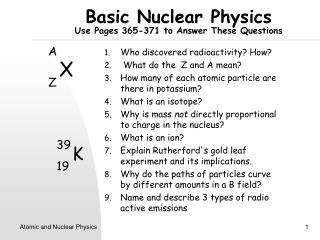DownloadDownload PresentationBasic Nuclear Physics Use Pages 365-371 to Answer These Questions

# Basic Nuclear Physics Use Pages 365-371 to Answer These Questions

Télécharger la présentation## Basic Nuclear Physics Use Pages 365-371 to Answer These Questions

- - - - - - - - - - - - - - - - - - - - - - - - - - - E N D - - - - - - - - - - - - - - - - - - - - - - - - - - -
##### Presentation Transcript

1. Basic Nuclear PhysicsUse Pages 365-371 to Answer These Questions A Z X Who discovered radioactivity? How? What do the Z and A mean? How many of each atomic particle are there in potassium? What is an isotope? Why is mass not directly proportional to charge in the nucleus? What is an ion? Explain Rutherford's gold leaf experiment and its implications. Why do the paths of particles curve by different amounts in a B field? Name and describe 3 types of radio active emissions 39 19 K Atomic and Nuclear Physics

2. Basic Nuclear PhysicsUse Pages 365-371 to Answer These Questions • What are the relative ionizing and penetrating abilities of the 3 nuclear emissions? • How is radioactivity measured? • What is half-life? • What is conserved in a nuclear reaction? • Complete: 22688Ra 22286Rn • Do you have a radioactive source in your home? Now try problems 1-8 on page 385 in your text. Atomic and Nuclear Physics

3. The Photoelectric EffectRef: page 332-338 • This occurs when light shines onto a piece of shiny metal and causes electrons to be given off. • U.V. light is needed for most metals. • Uses: ??? • What happens when? • The frequency of light is changed • The brightness of light is changed Atomic and Nuclear Physics

4. Photons • Packets or quanta of energy. • Fixed amount of energy • E  f  E=hf • h=plancks constant 6.63x10-34Js The Photoelectric EffectRef: page 332-338 • Investigating the Photoelectric Effect • Adjust the voltage so electrons are just stopped and the current is zero. • The voltage is noted for different frequencies of light. Atomic and Nuclear Physics

5. Investigating the Photoelectric EffectRef:P336 • fois the threshold frequency, below this value no electrons are given off. • kinetic energy=voltage x charge • Ek=Ve= max Ek of emitted electrons • The graph can therefore be drawn as: V vs. f V f fo Ek vs. f Ek • The gradient of the graph is h Planck's constant •  is the work function or minimum amount of energy required for electrons to escape the surface. fo f Ek = hf -   Atomic and Nuclear Physics

6. Photoelectric Effect Problems 1) Which formula on your formula sheet refers to the photo electric effect. 2) What does hf , Ek and  refer to? 3) What two things would be different about the graph of Ek vs. f if a different metal were used? 4) What would be the same on the above graph if a different metal were used? Try text problems 1-11 page 347-348. Atomic and Nuclear Physics